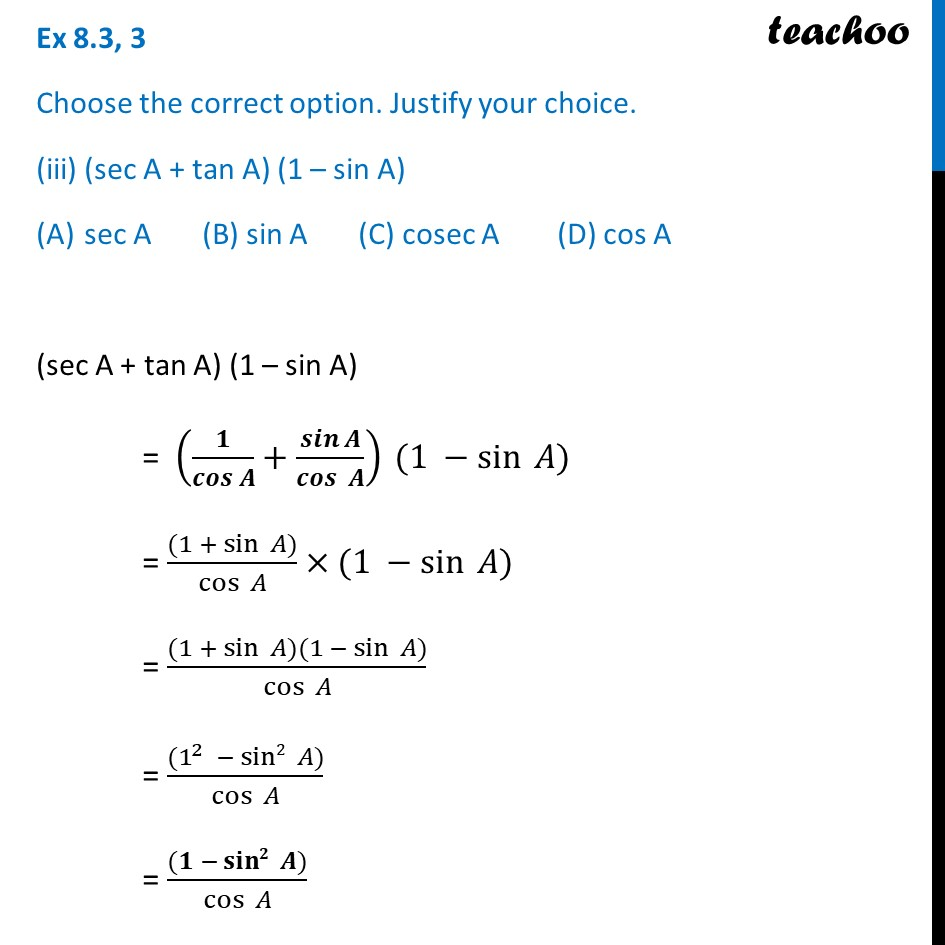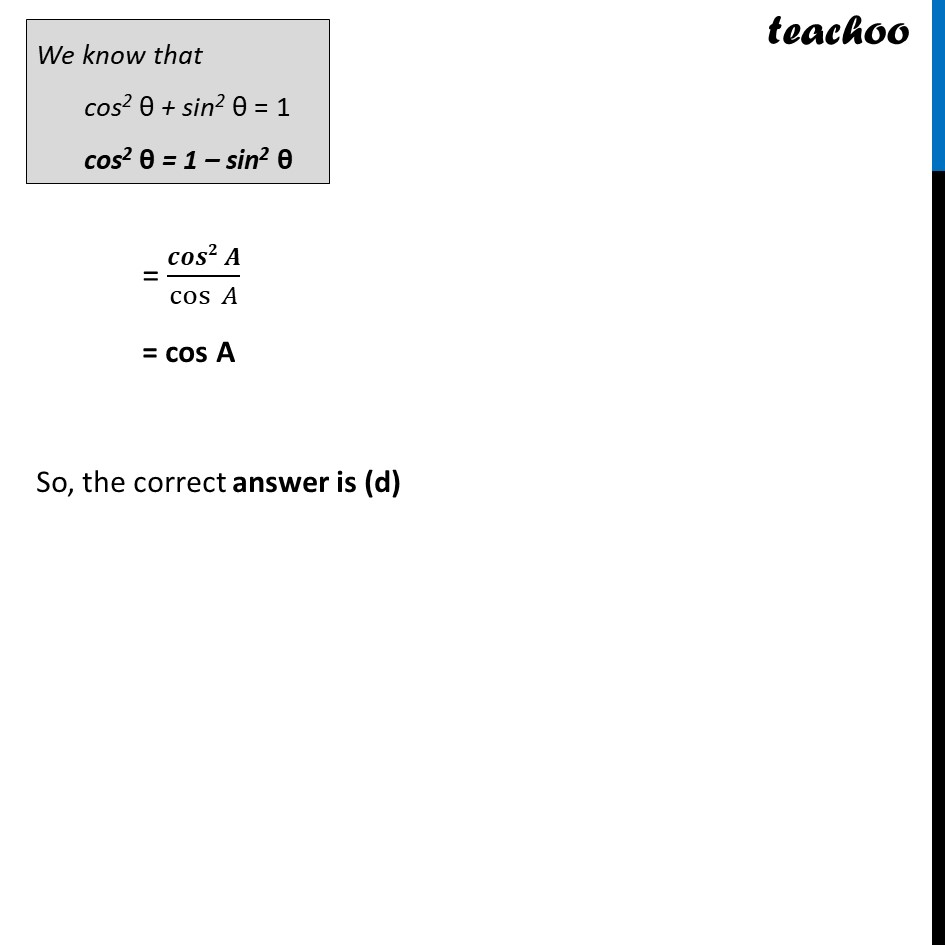Ex 8.3

Chapter 8 Class 10 Introduction to Trignometry
Serial order wiseLearn in your speed, with individual attention - Teachoo Maths 1-on-1 Class

### Transcript

Ex 8.3, 3 Choose the correct option. Justify your choice. (iii) (sec A + tan A) (1 – sin A) sec A (B) sin A (C) cosec A (D) cos A (sec A + tan A) (1 – sin A) = (𝟏/(𝒄𝒐𝒔 𝑨)+𝒔𝒊𝒏⁡𝑨/𝒄𝒐𝒔⁡〖 𝑨〗 ) (1 −sin⁡〖 𝐴)〗 = ((1 + sin⁡〖 𝐴)〗)/cos⁡〖 𝐴〗 ×(1 −sin⁡〖 𝐴)〗 = ((1 + sin⁡〖 𝐴)(1 −〖 sin〗⁡〖 𝐴)〗 〗)/cos⁡〖 𝐴〗 = ((1^2 −〖 sin2〗⁡〖 𝐴)〗)/cos⁡〖 𝐴〗 = ((𝟏 −〖 𝐬𝐢𝐧𝟐〗⁡〖 𝑨)〗)/cos⁡〖 𝐴〗 = (𝒄𝒐𝒔𝟐 𝑨)/cos⁡〖 𝐴〗 = cos A So, the correct answer is (d)